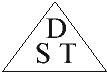# Motion

## Inertia

• Inertia is the tendency of a stationary object to remain at rest, or the tendency of a moving object to continue at the same speed.
• The heavier the object the greater is the inertia.
• Examples of Inertia
1. When a car brakes suddenly, the driver and passengers tend to keep going in the same direction and at the same speed as before braking. Seatbelts are therefore needed to stop them going through the windscreen.
1. It is more difficult to push-start a stationary truck than it is to push a small sedan.
2. It is more difficult to stop a moving truck than it is to stop a small sedan.

## Distance and Displacement

• Distance is a measurement of length (e.g. 50 km, 200 m). It is a scalar quantity.
• Displacement is distance in a particular direction (e.g. 50 km North, 200 m Up). It is a vector quantity.

## Speed and Velocity

• Speed is the rate of movement (e.g. 50 km/h, 200 m/s). It is a scalar quantity.
• Velocity is speed in a particular direction (e.g. 50 km/h north, 200 m/s to the right). It is a vector quantity.
• Speed and Velocity RulesSpeed (S) = Distance (D) Time (T)

Distance (D) = Speed (S) × Time (T)

 Time (T) = Distance (D) Speed (S)

 Average Speed = Total Distance Total Time

## Safe Driving

• Stopping Distance - When a driver sees a hazard ahead, he/she takes a short time to recognise what the hazard is, and then hits the brakes. The distance travelled in this time is called the reaction distance. Then the car comes to a stop a short distance after applying the brakes. This is called the braking distance.

Stopping distance = Reaction distance + Braking distance
• Factors Affecting Stopping Distance
1. Blood Alcohol Content (BAC) - Alcohol greatly affects a driver's ability.
2. Age and Experience - Older drivers tend to have slower reflexes. However, younger drivers lack skills of anticipation and avoidance.
3. Tyre Tread, Brake Condition and Road Conditions also affect a car's stopping ability.

## Acceleration

• Acceleration is the rate of change of speed.
When a car accelerates, it speeds up. When a car decelerates, it slows down.
• Acceleration Rule
 Acceleration (a) = Final velocity (v2) – Initial velocity (v1) Time (t)
• Example of Acceleration Rule

Question: A car starts from rest (initial velocity = 0 m/s) and speeds up to 50 m/s (final velocity) in 5 seconds. What is its acceleration?
 Answer: a = (v2 – v1) = (50 - 0) = 50 = 10 m/s2 t 5 5
• Earth's Gravitational Acceleration (g) is the acceleration of an object as it falls to earth at 9.8m/s. This means that an object that falls starts at 0m/s, and then every second, its speed increases by 9.8m/s.

## Momentum

• Momentum is a measure of motion of an object. It is measured in kilogram-metres per second (kg.m/s).
• Momentum Rule
 Momentum (kg.m/s) = Mass (kg) × Velocity (m/s) Momentum = m × v p = mv
• Example of the Momentum Rule

Question: While ice-skating, a man whose mass is 100 kg pushes away from the fence at a speed of 5 m/s. How much momentum does he have?
 Answer:   Momentum = mv = m × v = 100 × 5 = 500 kg.m/s